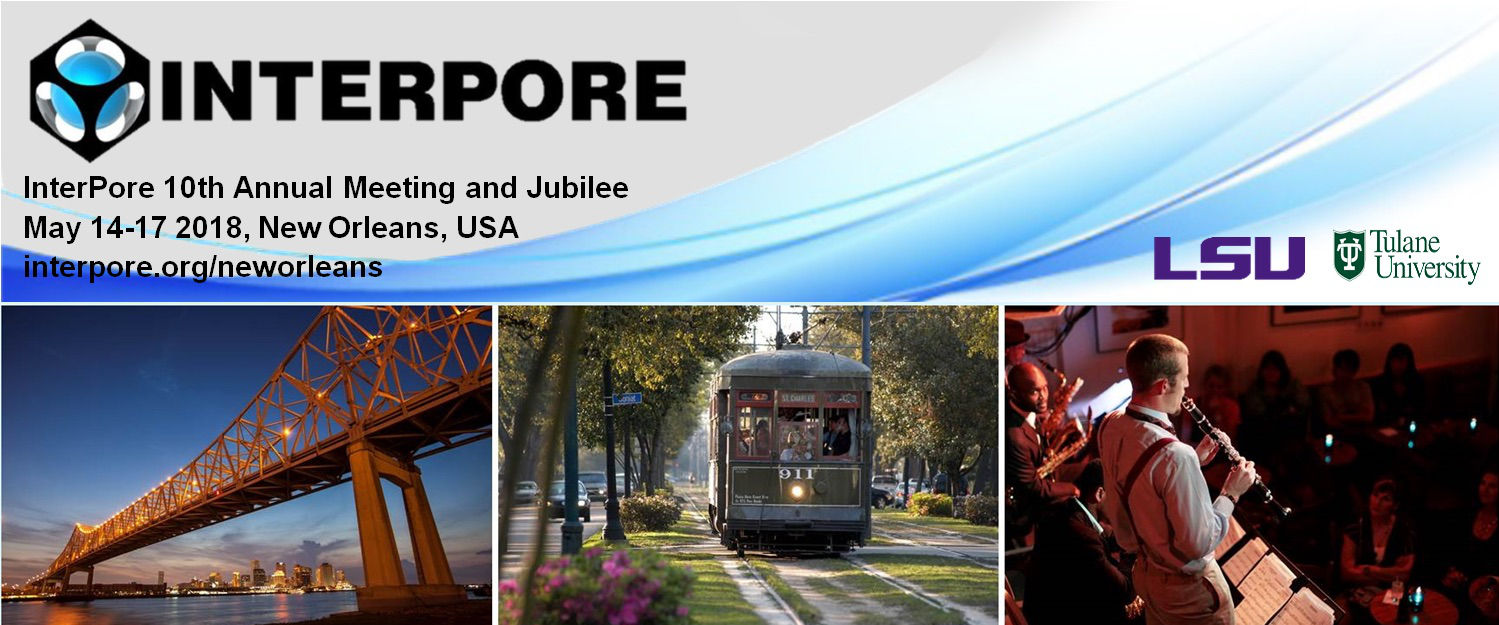#InterPore2018 New Orleans

14-17 May 2018
New Orleans
US/Central timezone

## From Red Cells to Soft Porous Lubrication

17 May 2018, 11:23
15m
New Orleans

#### New Orleans

Oral 20 Minutes MS 4.17: Transport through Soft Porous Media

### Speaker

Qianhong Wu (Villanova University)

### Description

In this paper, we report a novel experimental study to examine the lubrication theory for highly compressible porous media (Feng & Weinbaum, JFM, 422, 282, 2000), which was applied to the frictionless motion of red cells over the endothelial surface layer (ESL). The experimental setup consists of a running conveyer belt covered with a soft porous sheet, and an upper planar board, i.e. planing surface. The pore pressure generation was captured when the planing surface glides over the porous sheet. If the lateral leakage was eliminated, we found that the overall pore pressure’s contribution to the total lift, $f_{air}$≈80%, and the friction coefficient $η=0.0981$, when $U=5$ m/s, $L=0.381$ m, $λ=h_2/h_0=1$ and $k=h_2/h_1=3$, where $U$ is the relative velocity of the conveyor belt; $L$ is the length of the planing surface; $h_0$, $h_1$ and $h_2$ are the undeformed, leading edge and trailing edge porous layer thickness, respectively. $f_{air}$ increases with the increase in $U$, $λ$ and $L$, while decreases with the increase in $k$. $η$ decreases with the increase in $f_{air}$. If later pressure leakage exists, the pore pressure generation is reduced by nearly 90%. All the experimental results agreed well with the theoretical predictions. The study presented herein lays the foundation for applying soft porous media for new type of bearing with significantly reduced friction.